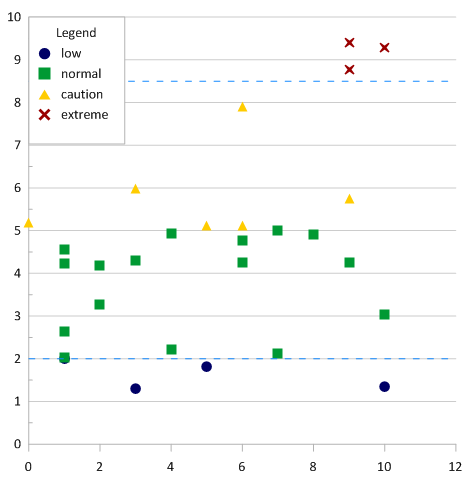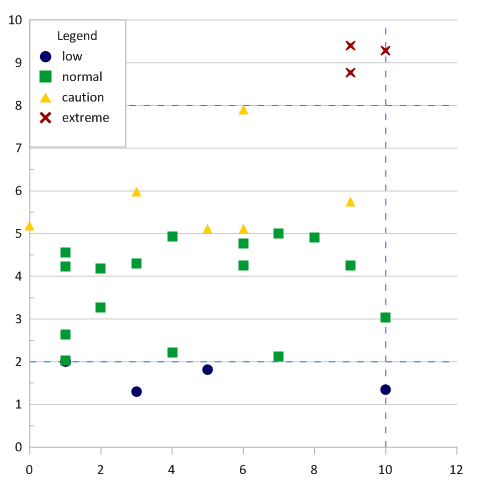Follow

# How can I get a line of constant value on my Grapher graph?

There are two methods in Grapher to add a line of constant value to your graph. The first is to add a function plot. The second is to add a grid line to an existing axis. Both methods are outlined below.

Function Plot:

To add a line of constant value using a function plot, you can use these steps:

1. Click anywhere on the existing graph to select it.
2. Click the Home | Add to Graph | Plot command ().
3. In the Select Plot Type dialog, select Y=F(X) Function Plot and click OK.
4. In the Choose Axes dialog, select the axes to use for the new constant value line and click OK. The plot is added using the default options.
5. Click on the Function Plot in the Object Manager to select it.
6. In the Property Manager, click on the Plot tab.
7. For a horizontal line, highlight the cos(X) text in the Y = F(X) = field and enter the desired value for the horizontal line. For instance, you might type 30, if you want the line displayed at Y=30.
8. For a vertical line,
1. Change the Type from Y=F(X) to X=F(Y).
2. Highlight the cos(Y) text in the X = F(Y) = field and enter the desired value for the vertical line. For instance, you might type 400, if you want the line displayed at X=400.
9. In the Property Manager, click the Data Limits tab.
10. Highlight the value 12 in the Last value field and enter the axis maximum value. For a horizontal line, this should be the X maximum value. For a vertical line, this should be the Y maximum value.
11. Highlight the zero in the First value field and enter the axis minimum value. For a horizontal line, this should be the X minimum value. For a vertical line, this should be the Y minimum value.

The line now extends the full length of the plot at the specified value. The line style and color can be changed on the Line tab in the Property Manager.Axis Grid Lines:

Axis grid lines may be easier to use if you have many horizontal or vertical lines that you want to display. It may also be easier if the axis limits will change. To draw lines of constant value using the axis grid lines:

1. Click on the existing worksheet or create a new worksheet.
2. In one column, enter the Y value for each horizontal line.
3. In another column, enter the X value for each vertical line.
4. Save the data file.
5. Click back to the Plot window.
6. For a horizontal line, click on the Y Axis to select it. For a vertical line, click on the X Axis to select it.
7. In the Property Manager, click on the Line tab.
8. Click the + next to the Grid Line Properties section, if needed.
9. Check the box in the Worksheet grid lines field.
10. Click the + next to the Worksheet Grid Line section.
11. Click in the Worksheet field and select the worksheet file that was saved in step 4.
12. Click in the Variable field and select the appropriate column. For horizontal lines, this is the column created in step 2. For vertical lines, this is the column created in step 3.

The line now extends the full length of the parallel axis. The line style and color can be changed in the Worksheet line properties section.Updated October 12, 2018